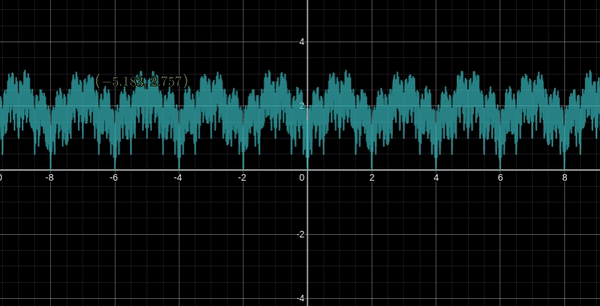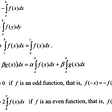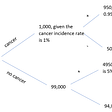# Derivatives

The formulas above shows the formula to find the derivative of a simple polynomial function. We start by taking the exponent n an multiplying it by our variable x. then we take the exponent n an subtract it by one.

# Product Rule

The product rule allows you to take the derivative of two different functions. Notice that the formula is f times g prime plus f prime times g. The prime symbol is our way of saying derivative. So f prime means the derivative of f.

# Chain Rule

The chain rule is used when we want to find the derivative of a function and a second function resting inside the function. Notice in the top function we move the exponent to the front of the function an then multiply that function by the derivative of the inner function.

Quotient Rule

The quotient rule has a lot in common with the product rule but instead we are dividing to functions rather then multiplying.

# Derivatives of trig functions

Derivatives of exponents

# Derivatives of logarithms

The top function is the formula to find the derivative of a natural logarithm

The lower s the derivative of a standard logarithm .

--

--

--

Love podcasts or audiobooks? Learn on the go with our new app.

## Building your own Time Machine## Visualising a nowhere differentiable everywhere continuous function## Fundamental concepts of calculus-Integrals## An Actual Introduction to Bayesian Statistics## Everybody claims to be me but only I can show them miracles of the universe.## Introduction to Strong Typicality## Learning what it takes to edit sentences.## Bayes Rule: Intuitive and Mathematical understanding